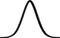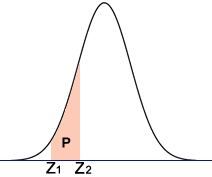# Z-score Calculator

Use this calculator to compute the z-score of a normal distribution.

 Raw Score, x Population Mean, μ Standard Deviation, σ## Z-score and Probability Converter

Please provide any one value to convert between z-score and probability. This is the equivalent of referencing a z-table.

## Result

Given Z = 1.96,

 P(xZ) = 0.024998P(0Z) = 0.049996Z-score, Z Probability, P(xZ) Probability, P(0 to Z or Z to 0) Probability, P(-ZZ)## Probability between Two Z-scoresUse this calculator to find the probability (area P in the diagram) between two z-scores.

 Left Bound, Z1 Right Bound, Z2RelatedStandard Deviation Calculator

### What is z-score?

The z-score, also referred to as standard score, z-value, and normal score, among other things, is a dimensionless quantity that is used to indicate the signed, fractional, number of standard deviations by which an event is above the mean value being measured. Values above the mean have positive z-scores, while values below the mean have negative z-scores.

The z-score can be calculated by subtracting the population mean from the raw score, or data point in question (a test score, height, age, etc.), then dividing the difference by the population standard deviation:

z =
 x - μ σ

where x is the raw score, μ is the population mean, and σ is the population standard deviation. For a sample, the formula is similar, except that the sample mean and population standard deviation are used instead of the population mean and population standard deviation.

The z-score has numerous applications and can be used to perform a z-test, calculate prediction intervals, process control applications, comparison of scores on different scales, and more.

### Z-table

A z-table, also known as a standard normal table or unit normal table, is a table that consists of standardized values that are used to determine the probability that a given statistic is below, above, or between the standard normal distribution. A z-score of 0 indicates that the given point is identical to the mean. On the graph of the standard normal distribution, z = 0 is therefore the center of the curve. A positive z-value indicates that the point lies to the right of the mean, and a negative z-value indicates that the point lies left of the mean. There are a few different types of z-tables.

The values in the table below represent the area between z = 0 and the given z-score.

Z Table from Mean (0 to Z)
 z 0 0.01 0.02 0.03 0.04 0.05 0.06 0.07 0.08 0.09 0 0 0.00399 0.00798 0.01197 0.01595 0.01994 0.02392 0.0279 0.03188 0.03586 0.1 0.03983 0.0438 0.04776 0.05172 0.05567 0.05962 0.06356 0.06749 0.07142 0.07535 0.2 0.07926 0.08317 0.08706 0.09095 0.09483 0.09871 0.10257 0.10642 0.11026 0.11409 0.3 0.11791 0.12172 0.12552 0.1293 0.13307 0.13683 0.14058 0.14431 0.14803 0.15173 0.4 0.15542 0.1591 0.16276 0.1664 0.17003 0.17364 0.17724 0.18082 0.18439 0.18793 0.5 0.19146 0.19497 0.19847 0.20194 0.2054 0.20884 0.21226 0.21566 0.21904 0.2224 0.6 0.22575 0.22907 0.23237 0.23565 0.23891 0.24215 0.24537 0.24857 0.25175 0.2549 0.7 0.25804 0.26115 0.26424 0.2673 0.27035 0.27337 0.27637 0.27935 0.2823 0.28524 0.8 0.28814 0.29103 0.29389 0.29673 0.29955 0.30234 0.30511 0.30785 0.31057 0.31327 0.9 0.31594 0.31859 0.32121 0.32381 0.32639 0.32894 0.33147 0.33398 0.33646 0.33891 1 0.34134 0.34375 0.34614 0.34849 0.35083 0.35314 0.35543 0.35769 0.35993 0.36214 1.1 0.36433 0.3665 0.36864 0.37076 0.37286 0.37493 0.37698 0.379 0.381 0.38298 1.2 0.38493 0.38686 0.38877 0.39065 0.39251 0.39435 0.39617 0.39796 0.39973 0.40147 1.3 0.4032 0.4049 0.40658 0.40824 0.40988 0.41149 0.41308 0.41466 0.41621 0.41774 1.4 0.41924 0.42073 0.4222 0.42364 0.42507 0.42647 0.42785 0.42922 0.43056 0.43189 1.5 0.43319 0.43448 0.43574 0.43699 0.43822 0.43943 0.44062 0.44179 0.44295 0.44408 1.6 0.4452 0.4463 0.44738 0.44845 0.4495 0.45053 0.45154 0.45254 0.45352 0.45449 1.7 0.45543 0.45637 0.45728 0.45818 0.45907 0.45994 0.4608 0.46164 0.46246 0.46327 1.8 0.46407 0.46485 0.46562 0.46638 0.46712 0.46784 0.46856 0.46926 0.46995 0.47062 1.9 0.47128 0.47193 0.47257 0.4732 0.47381 0.47441 0.475 0.47558 0.47615 0.4767 2 0.47725 0.47778 0.47831 0.47882 0.47932 0.47982 0.4803 0.48077 0.48124 0.48169 2.1 0.48214 0.48257 0.483 0.48341 0.48382 0.48422 0.48461 0.485 0.48537 0.48574 2.2 0.4861 0.48645 0.48679 0.48713 0.48745 0.48778 0.48809 0.4884 0.4887 0.48899 2.3 0.48928 0.48956 0.48983 0.4901 0.49036 0.49061 0.49086 0.49111 0.49134 0.49158 2.4 0.4918 0.49202 0.49224 0.49245 0.49266 0.49286 0.49305 0.49324 0.49343 0.49361 2.5 0.49379 0.49396 0.49413 0.4943 0.49446 0.49461 0.49477 0.49492 0.49506 0.4952 2.6 0.49534 0.49547 0.4956 0.49573 0.49585 0.49598 0.49609 0.49621 0.49632 0.49643 2.7 0.49653 0.49664 0.49674 0.49683 0.49693 0.49702 0.49711 0.4972 0.49728 0.49736 2.8 0.49744 0.49752 0.4976 0.49767 0.49774 0.49781 0.49788 0.49795 0.49801 0.49807 2.9 0.49813 0.49819 0.49825 0.49831 0.49836 0.49841 0.49846 0.49851 0.49856 0.49861 3 0.49865 0.49869 0.49874 0.49878 0.49882 0.49886 0.49889 0.49893 0.49896 0.499 3.1 0.49903 0.49906 0.4991 0.49913 0.49916 0.49918 0.49921 0.49924 0.49926 0.49929 3.2 0.49931 0.49934 0.49936 0.49938 0.4994 0.49942 0.49944 0.49946 0.49948 0.4995 3.3 0.49952 0.49953 0.49955 0.49957 0.49958 0.4996 0.49961 0.49962 0.49964 0.49965 3.4 0.49966 0.49968 0.49969 0.4997 0.49971 0.49972 0.49973 0.49974 0.49975 0.49976 3.5 0.49977 0.49978 0.49978 0.49979 0.4998 0.49981 0.49981 0.49982 0.49983 0.49983 3.6 0.49984 0.49985 0.49985 0.49986 0.49986 0.49987 0.49987 0.49988 0.49988 0.49989 3.7 0.49989 0.4999 0.4999 0.4999 0.49991 0.49991 0.49992 0.49992 0.49992 0.49992 3.8 0.49993 0.49993 0.49993 0.49994 0.49994 0.49994 0.49994 0.49995 0.49995 0.49995 3.9 0.49995 0.49995 0.49996 0.49996 0.49996 0.49996 0.49996 0.49996 0.49997 0.49997 4 0.49997 0.49997 0.49997 0.49997 0.49997 0.49997 0.49998 0.49998 0.49998 0.49998